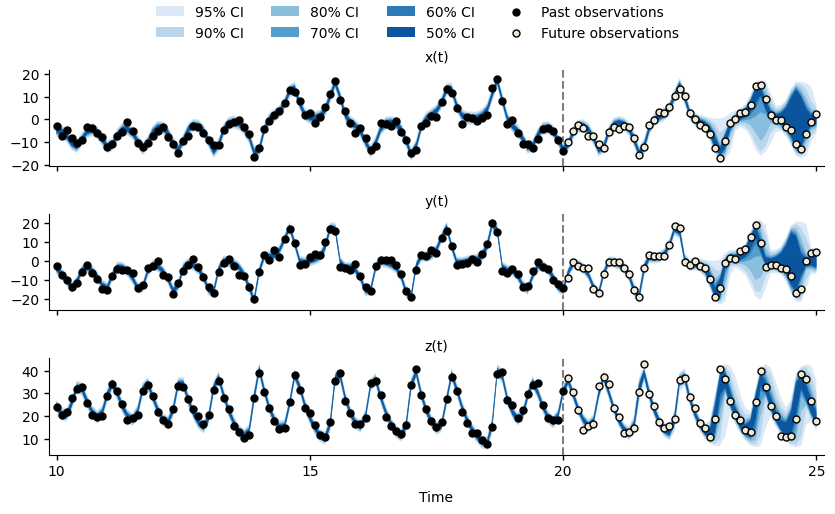# Bootstrap particle filter for Python¶

Welcome to the pypfilt documentation. This package implements a bootstrap particle filter that can be used for recursive Bayesian estimation and forecasting.

If there is a system or process that can be:

• Described (modelled) with mathematical equations; and

• Measured repeatedly in some (noisy) way.

Then you can use pypfilt to estimate the state and/or parameters of this system.Forecasts for $$x(t)$$, $$y(t)$$, and $$z(t)$$ of the Lorenz63 system at time $$t=20$$.¶

The Getting Started tutorial

Shows how to build a simulation model, generate simulated observations from this model, fit the model to these observations, and generate the example forecasts, above.

The Key Concepts guide

Introduces the various pypfilt components and how they fit together.

Note

This guide is currently incomplete.

The How-to Guides

Demonstrates how to solve particular problems.

Note

These guides are currently incomplete.

The Scenario Settings guide

Explains the settings that can be defined in a scenario file.

The API documentation

Presents the details of each pypfilt component and is likely only of interest if you need to develop your own components.

The code is distributed under the terms of the BSD 3-Clause license (see LICENSE), and the documentation is distributed under the terms of the Creative Commons BY-SA 4.0 license.